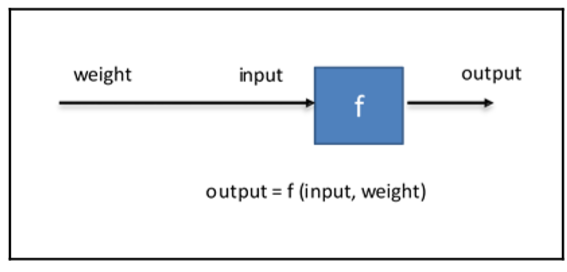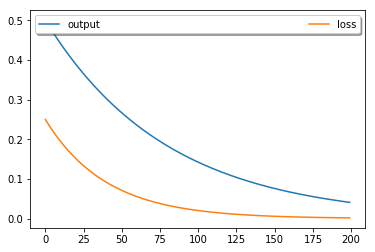• windows10
• python3.6
• jupyter notebook
• tensorflow 1.x

### 什么是人工神经网络$$A_1 = sigmoid(W_1 X)$$

$W_1$代表的是输入层和隐藏层之间每个神经元两两连接的线的权重, $A_1$表示图中的$[a_4, a_5, a_6, a_7]$, $sigmoid$表示激活函数, 它就是一个函数, 如果不知道可以先不理会. 从上图中, 你可以看出, $W_1$的形状是 4x3, 因为隐层是4个神经元, 输入层是3个神经元. 如果你没有学过线性代数, 那么你学习机器学习还是很有难度的, 但是没关系, 我这里列出计算隐层第一个神经元激活量$a_4$的公式:

$$a_4 = sigmoid(x_1 * a_{41} + x_2 * a_{42} + a_{43})$$

$$\hat Y = sigmoid(W_2 A_1)$$

### 世界上最简单的一个神经网络### 赞助